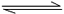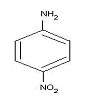Visitors Online: 0 | Monday 09th December 2019CBSE Guess > Papers > Important Questions > Class XI > 2013 > Chemistry By Mr. Sachin Prajapati CBSE CLASS XI
Chemistry Explain why 0-nitrophenol has a lower boiling point than p-nitrophenol? Why alkali metals used in photoelectric cells? Due to which compound, ozone depletion is caused in Antarctica? Which of the element among B, Al, C and Si :- Has the highest first ionization enthalpy? Has the most negative electron gain enthalpy? Write the atomic number of the element present in the third period and seventeenth group of the periodic table. Out of the elements Cr (Z-24), Mg (Z=12), and Fe (Z=26), identify the element with five electrons in 3d sub shell. Critical temperature of ammonia and carbon dioxide are 405.5 K and 304.10 K respectively. Which these gases will liquefy first when you start cooling from 500K to their critical temperature An element ‘A’ belongs to group 2 of the periodic table. It shows anomalous behavior from the rest of the elements of its group. It shows a diagonal relationship with another element ‘B’. Chlorides of both ‘A’ and ‘B’ have bridged structure in vapour phase. Identify A and B and draw the structures of their respective chlorides. Calculate the mass percent of different elements in sodium sulphate (Na2SO4). Why does the rain water normally have a pH of about 5.6? When does it become acid rain? The threshold frequency for the ejection of electron from a metal is 5 X 1014 s-1. Will the photon of radiation having energy 3.0 X 10-19 J give photo electric effect or not? A metal ‘X’ is present in chlorophyll. Identify the metal ‘X’. write the reaction of this metal with N2. An orbital has n=3. What are the possible values of l  and m l ? Explain the hybridization of PCl5 molecule. Why PCl5 on decomposition gives PCl3 and Cl2 ? Consider the chemical reaction: 2SO2 (g) + O2 (g)2SO3 (g) +189.4 KJ. Indicate the direction in which the equilibrium will shift when:-Temperature is increased. Pressure is increased. Concentration of SO2 is increased. Write the IUPAC names of the following compound:- For the reaction NH4Cl(s) → NH3 (g)+ HCl(g)  at 250C, enthalpy change ∇H =+177 kJmol-1and entropy change ∇S = +285JK-1mol-1 .Calculate free energy change  ∇G at 250 C and predict whether the reaction is spontaneous or not. Explain giving reasons :- Boron does not form B3+ ions. B-Cl bond has a dipole moment but BCl3 has zero dipole moment. Aluminium is used to make transmission cables. Alkali metals impart colour to the flame. Second ionization enthalpy of Na is higher than Mg. Which of the two is bigger in size and why? Cl or Cl- What is the conjugate base of HCO3- and H2O ? Why are metallic hydrides used for storing hydrogen? Name the two methods for estimation of nitrogen. Yellow light emitted from a sodium lamp has a wavelength (.) of 580 nm. Calculate frequency (.) and wave number of the yellow light? Calculate the oxidation number of Mn in K2MnO4 and N in HNO3. Compound ‘A’ with the molecular formula C5H8 reacts with hydrogen in the presence of Lindlar’s catalyst to form a compound ‘B’ with the molecular formula C5H10. ‘A’ on reacting with sodium in liquid ammonia forms a compound ‘C’ with same molecular formula as that of ‘B’. Identify A, B and C. Also write the chemical reactions involved. Write the general electronic configuration of p-block and f-block elements. Which of the following free radicals is most stable and why? CH2CH3